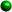Homework Tips for the WeekFactoring Trinomials The signs are key. Look at the sign of the third term.If it is positive, the signs in your answer will be the same, either both plus or both minus depending on the middle term.If it is negative, the signs in your answer will be different. If there is NO coefficient in the first term, it is an easier one where by listing the factors of the third term we can see which factors add or subtract to give us the middle term. Always check by FOIL!If there is a coefficient in the first term, the problem is a little harder and we must factor by trial and error. Don't get frustrated.It is factoring by trial and error. Use a pencil with an eraser! Sometimes you can get so involved with the numbers, you forget to check the signs, be careful. For examples and further explanation especially the trinomials with a coefficient in the first term see: Student Tutorials- Factoring Trinomials Simplifying Algebraic Fractions If the numerator or denominator can be factored... factor it. Determine whether there is a greatest common factor, or the expression is an example of the difference of two squares, or if it is a trinomial to be factored. Any expression over itself will equal one.Cancel common factors in the numerator and denominator. Remember, we can only cancel factors, not terms in division! Multiplying & Dividing Algebraic Fractions Remember in division to invert the fraction to the right and proceed as in multiplication.We can cancel any common factor in a numerator with any common factor in a denominator. First, if anything can be factored, factor it! Look at your answer and always ask yourself ,"Is there anything else I can do? Can anything else be simplified further or factored?"